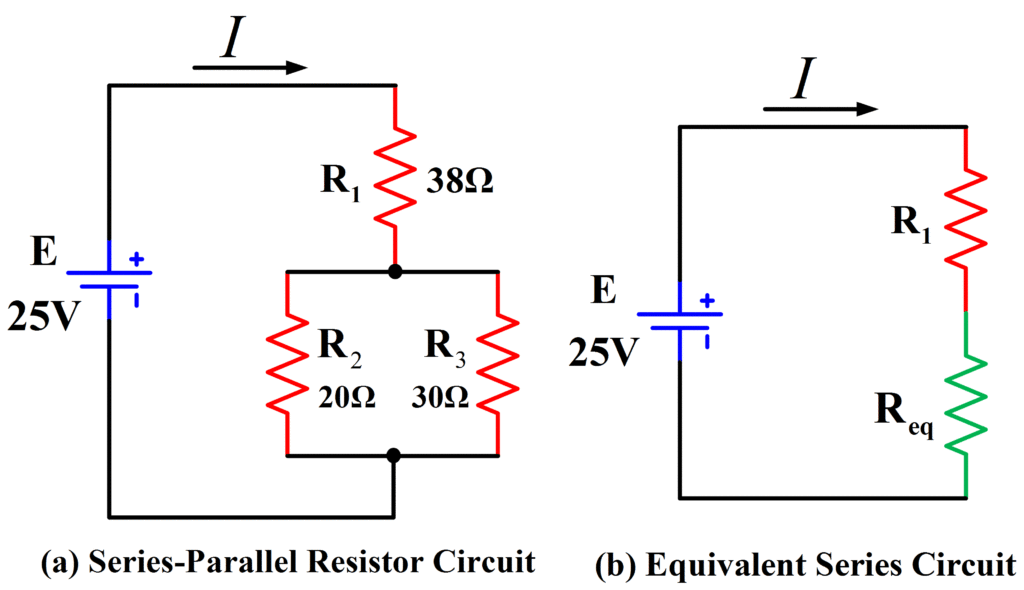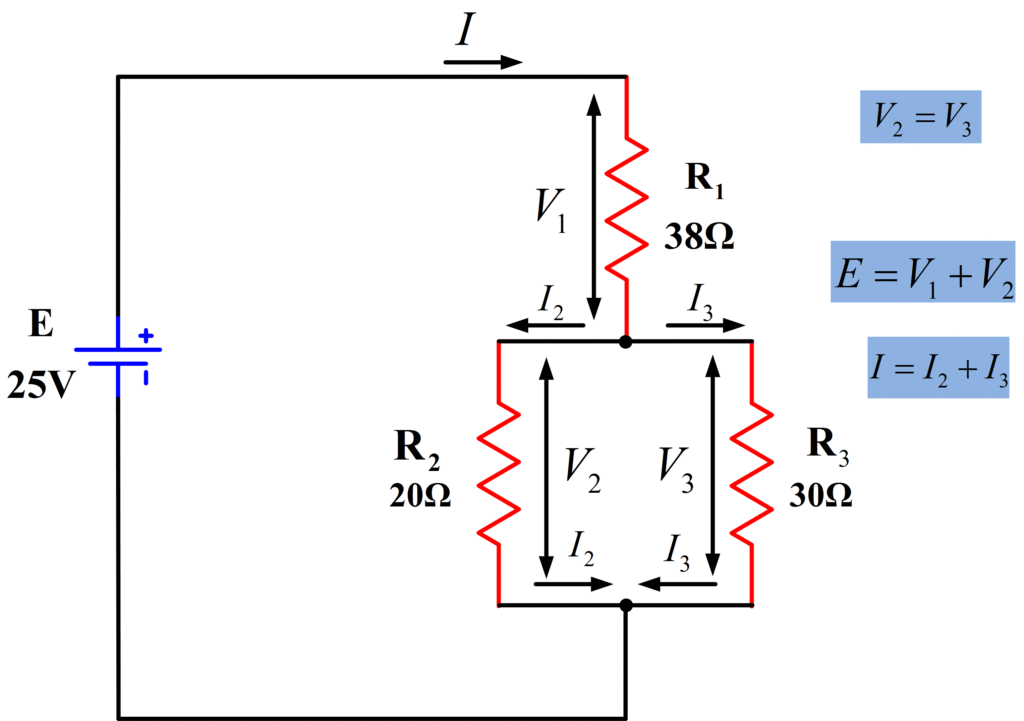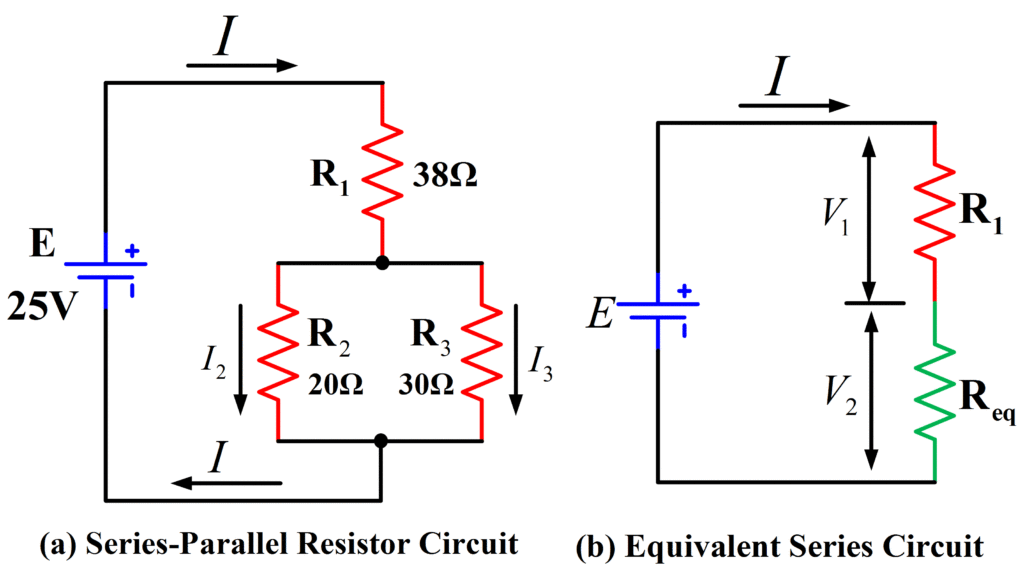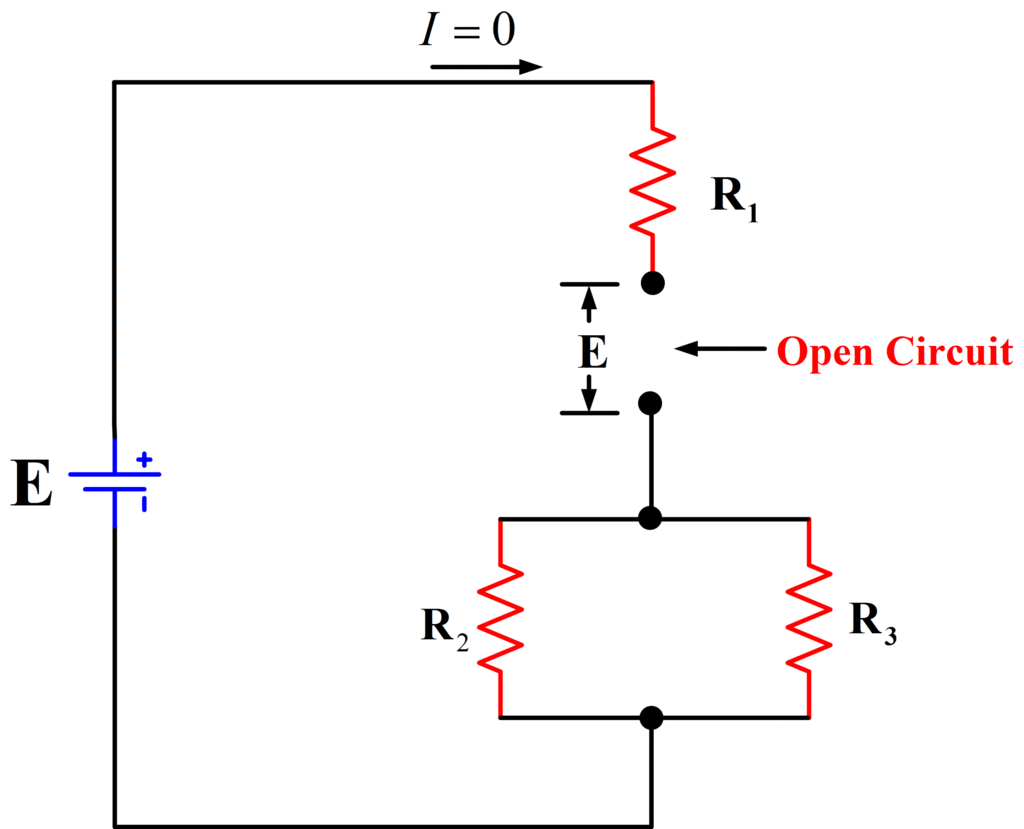Home / Basic Electrical / Series Parallel Circuit | Series Parallel Circuit Examples

# Series Parallel Circuit | Series Parallel Circuit Examples

Want create site? Find Free WordPress Themes and plugins.

## Series-Parallel Circuit Definition

Not all circuits are simple series or parallel arrangements. Many are combinations of parallel resistors connected in series with other resistors or combined with other parallel groups. These can be described as a series-parallel circuit.

The simplest approach to analyzing a series-parallel circuit is to resolve each purely series group into its single equivalent resistance and to resolve each parallel group of resistors into its equivalent resistance. The process is repeated as many times as necessary.

As in all types of circuits, open-circuit and short-circuit conditions affect the currents and voltage drops throughout the circuit.

## Simple Series-Parallel Circuit

Series-Parallel resistor circuits consist of combinations of series-connected and parallel-connected resistors. Figure 1 shows a circuit diagram of a very simple three-resistor series-parallel circuit. Resistors R2 and R3 are seen to be connected in parallel, and resistor R1 is in series with the parallel combinations of R2 and R3. The circuit currents vary from branch to branch, and the component voltage drops depend on the branch currents and on the component resistances. The supply current depends on the supply voltage and on the circuit resistance offered to the voltage source. Kirchhoff’s voltage and current laws are applied for analyzing series-parallel circuits.Fig.1: Circuit Diagram of Series-Parallel Circuit

## Series-Parallel Equivalent Circuits

In the circuit shown in figure 2 (a), resistors R2 and R2 are in parallel, and together they are in series with R1. The level of current taken from the supply is easily calculated if R2 and R3 are first replaced with their equivalent resistance (R2||R3) as illustrated in figure 2(b). The circuit now becomes a simple two-resistor series circuit.Fig.2: Series-Parallel Circuit and Equivalent Circuit

## Series-Parallel Circuit Example 1

Calculate the current drawn from the supply in the circuit shown in figure 2 (a).

Solution

Draw the equivalent circuit as in figure 2 (b) with

${{R}_{eq}}={{R}_{2}}||{{R}_{3}}$

To compute equivalent resistance:

${{R}_{eq}}=\frac{{{R}_{2}}*{{R}_{3}}}{{{R}_{2}}+{{R}_{3}}}=\frac{20*30}{20+30}=12\Omega$

$I=\frac{E}{{{R}_{1}}+{{R}_{eq}}}=\frac{25}{38+12}=0.5A$

In figure 3 (a), another series-parallel resistor combination is shown. In this case, the circuit is reduced to a simple parallel circuit when R2 and R3 are replaced by their equivalent resistance. [See Figure 3(b)]Fig.3: Series-Parallel Circuit and its Equivalent Circuit

## Series-Parallel Circuit Example 2

Determine the level of the supply current for the circuit shown in figure 3 (a).

Solution

Draw the equivalent circuit as in figure 3(b).

${{R}_{eq}}={{R}_{2}}+{{R}_{3}}=35+40=75\Omega$

R1 and Req are in parallel:

$R={{R}_{1}}||{{R}_{eq}}$

To compute equivalent resistance:

$R=\frac{{{R}_{1}}*{{R}_{eq}}}{{{R}_{1}}+{{R}_{eq}}}=\frac{50*75}{50+75}=30\Omega$

$I=\frac{E}{R}=\frac{75}{30}=2.5A$

## Current in Series-Parallel Circuit

The circuit of figure 2 (a) is reproduced in figure 4 with the branch currents and voltages identified. It is seen that the supply current flows through resistor R1 and that it splits up into I2 and I3 in order to flow through R2 and R3. Returning to the supply negative terminal, the current is once again I. it is seen that

$I={{I}_{2}}+{{I}_{3}}$Fig.4: Current and Voltage in Series-Parallel Circuit

Similarly, the supply current splits up between the resistors in figure 5, which is a reproduction of a circuit shown in figure 3 (a). Here I1 flows through R1, and I2 flows through R2 and R3, and the supply current is

$I={{I}_{1}}+{{I}_{2}}$Fig.5: Currents and Voltages in Series-Parallel Circuit

In each of these cases, the current through the individual resistors can be calculated easily using current-divider rule.

## Voltage drops in Series-Parallel Circuit

As always, the voltage drop across any resistor is the product of the resistance value and the current through the resistor. In figure 4,

${{V}_{1}}=I{{R}_{1}}$

And

${{V}_{2}}={{I}_{2}}{{R}_{2}}={{V}_{3}}={{I}_{3}}{{R}_{3}}$

Also,

$E={{V}_{1}}+{{V}_{2}}$

Similarly, in figure 5,

${{V}_{1}}={{I}_{1}}{{R}_{1}}$

${{V}_{2}}={{I}_{2}}{{R}_{2}}$

${{V}_{3}}={{I}_{2}}{{R}_{3}}$

$E={{V}_{1}}={{V}_{2}}+{{V}_{3}}$

## Series-Parallel Circuit Example 3

Using the voltage divider theorem, analyze the circuit in figure (a) below to determine the resistor voltage drops and the branch currents.Fig. Series-Parallel Circuit Example

Solution

${{R}_{eq}}={{R}_{2}}||{{R}_{3}}$

${{R}_{eq}}=\frac{{{R}_{2}}*{{R}_{3}}}{{{R}_{2}}+{{R}_{3}}}=\frac{20*30}{20+30}=12\Omega$

For voltage divider R1 and Req, as shown in figure (b) above:

${{V}_{2}}=E*\frac{{{R}_{eq}}}{{{R}_{1}}+{{R}_{eq}}}=25*\frac{12}{38+12}=6V$

${{V}_{1}}=E*\frac{{{R}_{1}}}{{{R}_{1}}+{{R}_{eq}}}=25*\frac{38}{38+12}=19V$

For branch currents:

$I=\frac{{{V}_{1}}}{{{R}_{1}}}=\frac{19}{38}=0.5A$

${{I}_{2}}=\frac{{{V}_{2}}}{{{R}_{2}}}=\frac{6}{20}=0.3A$

${{I}_{3}}=\frac{{{V}_{3}}}{{{R}_{3}}}=\frac{6}{30}=0.2A$

## Open-Circuit and Short-circuit in a Series-Parallel Circuit

The effect of an open-circuit or short-circuit condition on a series-parallel circuit depends on just where in the circuit the fault occurs. Consider figure 6, where an open-circuit is shown at the end of R1. This has the same effect as an open-circuit in the supply line so that all current levels are zero. Also, because the currents are zero, there are no voltage drops across resistors, and consequently all of the supply voltage E appears across the open-circuit.Fig.6: Open-Circuit at Resistor R1

An open-circuit in one branch of a series-parallel circuit usually alters the current levels in several branches of the circuit.

In the case of an open-circuit at one end of the parallel resistors, as shown in figure 7, I2 goes to zero. The current through R1 and R2 is now equal to the supply current and is calculated as

$I=\frac{E}{{{R}_{1}}+{{R}_{2}}}$

Also, because there is no current through R3, there is no voltage drop across it, and the voltage at the open circuit is equal to V2.Fig.7: Open-Circuit at Resistor R3

For the short circuit condition shown in figure 8, the resistance between the terminals of R1 is effectively zero. Therefore, the supply voltage appears across R2 and R3 in parallel. This gives a supply current of

$I=\frac{E}{{{R}_{2}}||{{R}_{3}}}$

And the branch currents are

${{I}_{2}}=\frac{E}{{{R}_{2}}}$

And

${{I}_{3}}=\frac{E}{{{R}_{3}}}$

It is seen that the levels of current through R2 and R3 have been increased from the normal (before the short-circuit) condition. This could cause excessive power dissipation in the components if they have previously been operating near their maximum rating.Fig.8: Short-Circuit Across Resistor R1

A short-circuit in one branch of a series-parallel circuit usually alters the current levels in several branches of the circuit.

The short-circuit condition illustrated in figure 9 effectively reduces I2 and I3 to zero and increases the supply current to

$I=\frac{E}{{{R}_{1}}}$

Obviously, the current through R1 is now greater than normal, and again power dissipation might present a problem.Fig.9: Short-Circuit Across Resistor R3

## Analyzing a Series-Parallel Circuit

Analysis procedure for series-parallel resistor circuits is as follow:

1. Draw a circuit diagram identifying all components by number and showing all currents and resistor voltage drops.
2. Convert all series branches of two or more resistors into a single equivalent resistance.
3. Convert all parallel combinations of two or more resistors into a single equivalent resistance.
4. Repeat procedures 2 and 3 until the desired level of simplification is achieved.

The final circuit should be straightforward series or parallel circuit, which can be analyzed in the normal way. Once the current through each equivalent resistance, or the voltage across it, is known, the original circuit can be used to determine individual resistor currents and voltages.

Did you find apk for android? You can find new Free Android Games and apps.